# Metadynamics Simulation of Cu Vacancy Diffusion on Cu(111) - Using PLUMED¶

Version: P-2019.03

In this tutorial you will learn how to setup and run metadynamics simulations in QuantumATK using the PlumedMetadynamics class and the PLUMED plugin. It will cover setting up a simulation to explore the free-energy landscape associated with the diffusion of a Cu vacancy on Cu(111), how to setup a metadynamics calculation, and how to analyze the results.

## Introduction¶

Metadynamics [LP02] is a powerful method based on molecular dynamics to explore multidimensional free energy surfaces (FESs). It allows one to reconstruct the FES of a system as a function of a number of collective variables (CVs) which are based on the microscopic coordinates of the system.

In metadynamics, an additional bias potential is added to the system at regular intervals during the simulation time. This allows the system to escape deep free-energy minima and overcome high-energy barriers, thereby exploring efficiently the entire free-energy surface. Within materials science, the method has been used to investigate crystal polymorphism, solid-liquid interfaces, and chemical reactions in solids and surfaces. Here, you will apply it to investigate the diffusion of a Cu vacancy on Cu(111).

QuantumATK implements metadynamics via the PLUMED plugin. In this tutorial, we will introduce the method briefly, explain how to setup a metadynamics script for the vacancy diffusion on Cu(111), and how to analyze the resulting free-energy surface.

## Theoretical Background¶

In metadynamics, an external bias potential is constructed in the phase space of a few selected degrees of freedom, $$S$$, generally called collective variables (CVs) [LP02]. $$S$$ is a set of $$d$$ functions of the microscopic coordinates $$R$$ of the system.

$S(R) = (S_1 (R), ..., S_d (R)).$

This potential is built as a sum of Gaussians deposited along the trajectory in the CVs space at time $$t$$. In its simplest version, the potential takes the form [BBP11]

$V(S,t) = \int_{0}^{t} dt^{'} \frac{W}{\tau} \exp\left( -\sum_{i=1}^{d} \frac{(S_i (R)-S_i({R}(t^{'} )))^2}{2\sigma_i^2} \right),$

where $$\tau$$ is the Gaussian deposition stride, $$\sigma_i$$ is the width of the Gaussian for the $$i$$-th CV, and $$W$$ is the height of the Gaussian. The effect of the bias potential is to push the system away from local minima and allow it to visit new regions of the phase space.

## Metadynamics Simulation of Cu Vacancy on Cu(111)¶

### Creating a Vacancy on Cu(111)¶

To create the Cu vacancy on Cu(111), you first need to make a Cu(111) surface.

In theBuilder, click on Add‣From Database and import ‘Copper‘. Then click on Builders ‣ Surface (Cleave) to create the Cu(111) surface, using the following settings:

• Set the Miller indices to $$\langle 1 1 1 \rangle$$
• Use the surface lattice shown in the image below:• Set the out-of-plane cell vector as ‘Non-periodic and normal (slab)
• Set the thickness to 6 layers and the vacuum gap to 10 ÅNext, create a vacancy by deleting the atom of the topmost layer with position $$(x,y) = (0,0)$$ (shown in red in the figure below):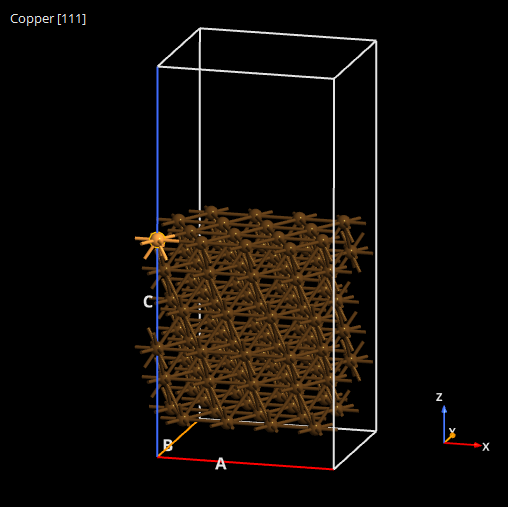Finally, click on Selection Tools ‣ Tags and tag the 4 lowermost Cu(111) layers. This tag will be used to fix them during the metadynamics simulation.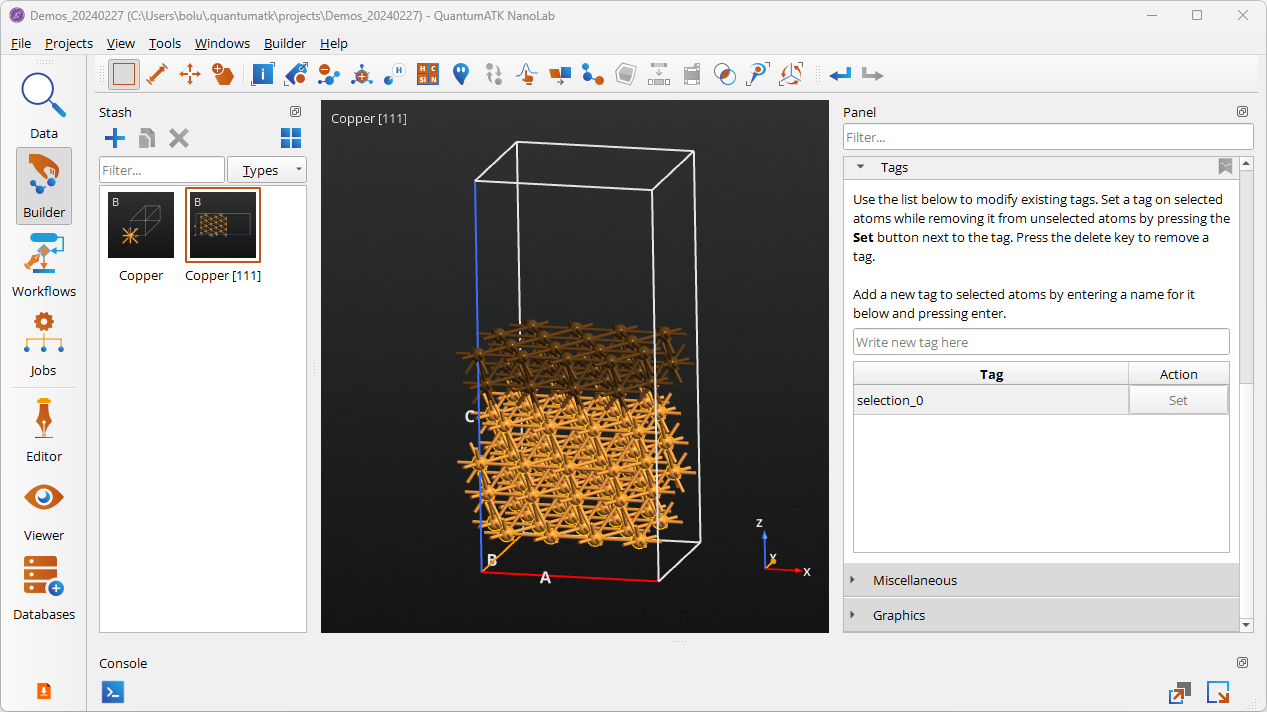Now you are ready to create the script to perform the metadynamics simulation.
Send the structure from the Stash to the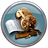Script Generator and setup the script as follows:1. Double click on theForceFieldCalculator block and use the ‘EAM_Cu_2001b‘ parameter set.1. Open the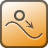MolecularDynamics block and set the simulation parameters as shown in the figure below:1. Finally, click on Add Constraints and fix the bottommost 4 layers of Cu(111), which have been previously tagged.Send the script to the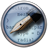Editor using the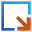icon in theScripter, and add the highlighted lines as shown below to include the PlumedMetadynamics class to perform the metadynamics calculation:

# -------------------------------------------------------------
# Calculator
# -------------------------------------------------------------

potentialSet = EAM_Cu_2001b()
calculator = TremoloXCalculator(parameters=potentialSet)
calculator.setVerletListsDelta(0.25*Angstrom)

bulk_configuration.setCalculator(calculator)
nlprint(bulk_configuration)
bulk_configuration.update()
nlsave('vacancy-hopping.hdf5', bulk_configuration)

# -------------------------------------------------------------
# Molecular Dynamics
# -------------------------------------------------------------

initial_velocity = MaxwellBoltzmannDistribution(
temperature=200.0*Kelvin,
remove_center_of_mass_momentum=True
)

method = Langevin(
time_step=1*femtoSecond,
reservoir_temperature=200*Kelvin,
friction=0.01*femtoSecond**-1,
initial_velocity=initial_velocity,
heating_rate=0*Kelvin/picoSecond,
)

fix_atom_indices_0 = [ 0,  1,  2,  3,  4,  5,  6,  7,  8,  9, 10, 11, 12,
13, 14, 15, 16, 17, 18, 19, 20, 21, 22, 23, 24, 25,
26, 27, 28, 29, 30, 31, 32, 33, 34, 35, 36, 37, 38,
39, 40, 41, 42, 43, 44, 45, 46, 47, 48, 49, 50, 51,
52, 53, 54, 55, 56, 57, 58, 59, 60, 61, 62, 63]

constraints = [FixAtomConstraints(fix_atom_indices_0)]

plumed_command = """\
UNITS LENGTH=A TIME=fs ENERGY=96.48533645956869
p: POSITION ATOM=81
METAD ARG=p.x,p.y SIGMA=0.025,0.025 HEIGHT=0.05,0.05 PACE=1000 LABEL=restraint
PRINT ARG=p.x,p.y,restraint.bias STRIDE=10 FILE=COLVAR
"""

configuration=bulk_configuration,
timestep=1.0*fs,
plumed_commands=plumed_command,
logfile='plumed.log',
)
md_trajectory = MolecularDynamics(
bulk_configuration,
constraints=constraints,
trajectory_filename='vacancy-hopping-trajectory.hdf5',
steps=10000000,
log_interval=1000,
post_step_hook=plumed_hook,
method=method
)

bulk_configuration = md_trajectory.lastImage()
nlsave('vacancy-hopping.hdf5', md_trajectory)


You can also download the input file from here: vacancy-hopping.py.

In the input above, the plumed_command section contains the input that will be passed directly to PLUMED [BBP11].

There are four command lines:

• UNITS: this line controls the units that will be used by PLUMED. Edit the lines as above, and the units of Å, femtoseconds, and eV will be used.

• p: This line is used to define the constrained atoms, in this case, the atom with index $$81$$.

Note

This value should be set according to the indexing used by PLUMED, where atom indexing starts from $$1$$, and not from $$0$$ as in QuantumATK. This value should therefore be set to “atom index $$+ 1$$”, where “atom index” is the index of the constrained atom.

We will look at the potential energy surface in the $$x$$- and $$y$$- axis using the gaussian function of the width ($$0.025$$) and height ($$0.05$$) on each axis.

• METAD: This line controls the type of metadynamics and the shape of Gaussians functions that will be used during the simulations:

• ARG: this flag is used to set the collective variables. In this case, we will use as collective variables the position of the atom $$p$$ along the Cartesian direction $$x\ (p.x)$$ and $$y\ (p.y)$$.
• SIGMA: this flag is used to set the value of the width of the Gaussian functions $$\sigma$$ used for each collective variable. In this case, we will use $$\sigma = 0.025$$ for both collective variables.
• HEIGHT: this flag is used to set the height of the Gaussian functions used for each collective variable. In this case, we will use a height of $$0.05$$ eV for both collective variables.
• PACE: this flag is used to set the intervals at which a new Gaussian function will be added. In this case, we will add a new Gaussian function every $$1000$$ MD steps.
• LABEL: this flag is used to set the name of the collective variables.
• PRINT: This line controls the format of the PLUMED output.

• ARG: this flag controls the variables that will be printed, in the present case $$p.x$$, $$p.y$$, and the bias.
• STRIDE: this indicates the printing interval. In the present case, $$10$$ MD steps.
• FILE: this line indicates the name of the output file.

If you would like to know more detailed information, we recommend you to look in the PLUMED documentation. These commands are passed to the PlumedMetadynamics class. The latter is passed to the MolecularDynamics class using a hook function.

Once you are done, send it to the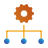Job Manager and run the simulation. It will take about 8 hours on 8 CPUs.

### Analyzing the Results¶

In addition to the standard QuantumATK output, at the end of the job you will obtain the files COLVAR and HILLS. These two files are the output of PLUMED and will be used to analyze the metadynamics simulation.

To plot the profile of the free energy $$\mathrm{F}$$ as a function of the collective variables $$\mathrm{CV\ 1}$$ and $$\mathrm{CV\ 2}$$, you first have to run the script extract_F.py as atkpython extract_F.py. Three output files will be produced:

• F_vs_cv1.dat contains the data for $$\mathrm{F}$$ vs. $$\mathrm{CV\ 1}$$.
• F_vs_cv2.dat contains the data for $$\mathrm{F}$$ vs. $$\mathrm{CV\ 2}$$.
• F_vs_cv1_cv2.dat contains the data for $$\mathrm{F}$$ vs. $$\mathrm{CV\ 1}$$ and $$\mathrm{CV\ 2}$$.

The map of the free energy profile $$\mathrm{F}$$ can be obtained by running the script plot_F_vs_cv1_cv2.py as:

atkpython F_vs_cv1_cv2.py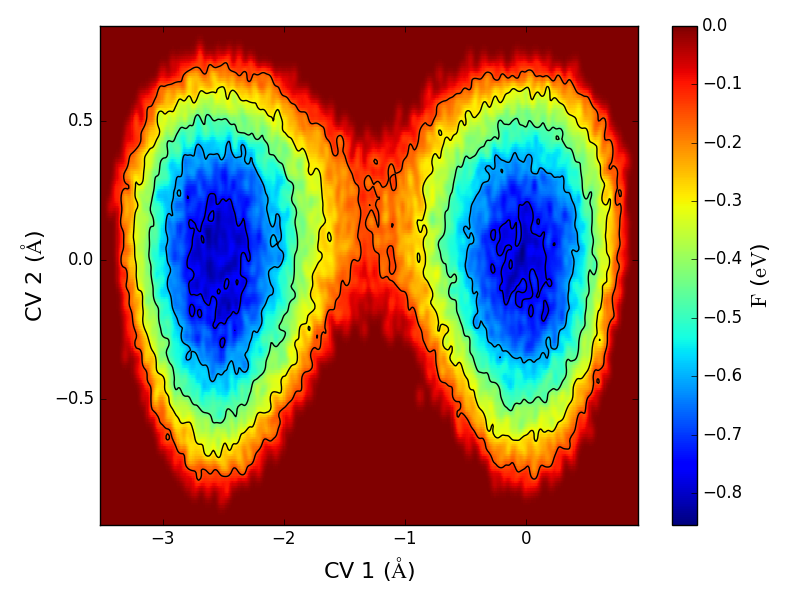The above plot shows a heat map of the free energy profile as a function of the collective variables $$\mathrm{CV\ 1}$$ and $$\mathrm{CV\ 2}$$. It can be seen that there are two minima on the free-energy surface, corresponding to the diffusion of the vacancy from one site to the neighboring one.

The evolution of the collective variables over the simulation time can be obtained by running the script cv1_cv2_vs_time.py as:

atkpython cv1_cv2_vs_time.pyThe resulting plot shows the evolution of $$\mathrm{CV\ 1}$$ (red) and $$\mathrm{CV\ 2}$$ (blue) as a function of the simulation time. It can be seen that $$\mathrm{CV\ 2}$$, which corresponds to the $$y$$ Cartesian coordinate, oscillates around a constant value ($$\mathrm{CV\ 2}$$ $$= 0$$ $$\mathrm{Å}$$), because both free energy minima occur at the same value of $$y$$. Conversely, the evolution of $$\mathrm{CV\ 1}$$, which corresponds to the x Cartesian coordinate, indicates that the simulation starts in the minimum on the left ($$\mathrm{CV\ 1}$$ $$= -2.5$$ $$\mathrm{Å}$$). The first minimum is filled until at approx. $$2.2$$ ns, and then the system moves to the second minimum ($$\mathrm{CV\ 1}$$$$= 0$$ $$\mathrm{Å}$$). After the second minimum is also filled, at around $$6$$ ns the system oscillates with equivalent probabilities between the two minima, until the simulation is completed.

The free energy barrier can also be analyzed by running the script barrier.py as:

atkpython barrier.pyThe results show that the barrier has a height of $$0.647$$ eV, in good agreement with the results in [KNB17].